# Resources tagged with: Functions and their inverses

Filter by: Content type:
Age range:
Challenge level:

There are 16 NRICH Mathematical resources connected to Functions and their inverses, you may find related items under Coordinates, Functions and Graphs.

Broad Topics > Coordinates, Functions and Graphs > Functions and their inverses### Equation Matcher

##### Age 16 to 18Challenge Level

Can you match these equations to these graphs?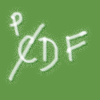### PCDF

##### Age 16 to 18Challenge Level

When can a pdf and a cdf coincide?### Real-life Equations

##### Age 16 to 18Challenge Level

Here are several equations from real life. Can you work out which measurements are possible from each equation?### Spaces for Exploration

##### Age 11 to 14

Alf Coles writes about how he tries to create 'spaces for exploration' for the students in his classrooms.### Area L

##### Age 16 to 18Challenge Level

By sketching a graph of a continuous increasing function, can you prove a useful result about integrals?### Function Pyramids

##### Age 16 to 18Challenge Level

A function pyramid is a structure where each entry in the pyramid is determined by the two entries below it. Can you figure out how the pyramid is generated?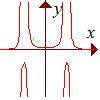### Rational Request

##### Age 16 to 18Challenge Level

Can you make a curve to match my friend's requirements?### Inverting Rational Functions

##### Age 16 to 18Challenge Level

Consider these questions concerning inverting rational functions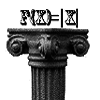### Maths Shop Window

##### Age 16 to 18Challenge Level

Make a functional window display which will both satisfy the manager and make sense to the shoppers### Curve Match

##### Age 16 to 18Challenge Level

Which curve is which, and how would you plan a route to pass between them?### Pitchfork

##### Age 16 to 18Challenge Level

Plot the graph of x^y = y^x in the first quadrant and explain its properties.### Double Time

##### Age 16 to 18Challenge Level

Crack this code which depends on taking pairs of letters and using two simultaneous relations and modulus arithmetic to encode the message.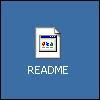##### Age 16 to 18Challenge Level

Decipher a simple code based on the rule C=7P+17 (mod 26) where C is the code for the letter P from the alphabet. Rearrange the formula and use the inverse to decipher automatically.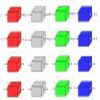### Machines

##### Age 7 to 11Challenge Level

What is happening at each box in these machines?### Modular Fractions

##### Age 16 to 18Challenge Level

We only need 7 numbers for modulus (or clock) arithmetic mod 7 including working with fractions. Explore how to divide numbers and write fractions in modulus arithemtic.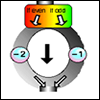### The Number Crunching Machine

##### Age 7 to 11Challenge Level

Put a number at the top of the machine and collect a number at the bottom. What do you get? Which numbers get back to themselves?